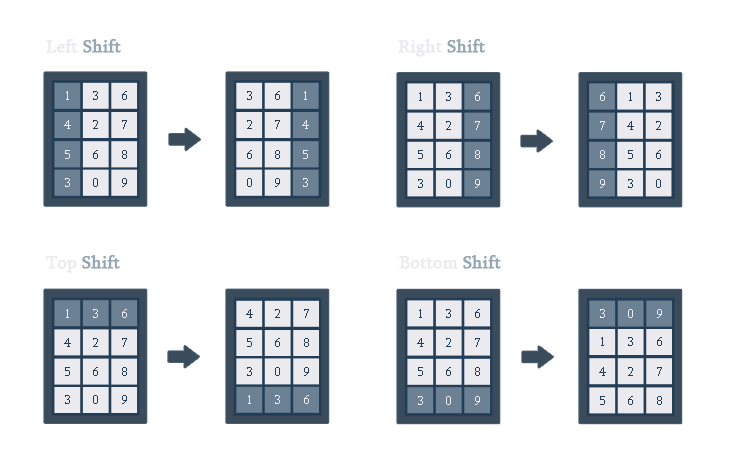### Problem Statement

Manao has an N x M matrix filled with digits. The rows in the matrix are consecutively numbered from 0 to N-1 starting from the top row and the columns are numbered from 0 to M-1 starting from the left column. He wants to perform several matrix shift operations in order to obtain a matrix with value in the upper left corner.

There are four types of matrix shifts: left, right, top and bottom. Performing the left shift on the matrix, we shift each of its columns one position to the left and put the first column in place of the last one. Similarly, right shift results in shifting each column to the right and placing the last one in place of the first. Top shift takes each row one position up and puts the first row in place of the last, and bottom shift shifts all the rows one position down and puts the last row in place of the first row. Note that if a matrix has only one row, then top and bottom shifts do not change the matrix in any way. Similarly, if a matrix has a single column, then right and left shifts have no effect.You are given a String[] matrix, where the j-th character of the i-th element is the digit in row i, column j. Compute and return the minimum possible total number of shifts that Manao should perform to accomplish his task. If there is no way to obtain a matrix with the upper left element equal to value, return -1 instead.

### Definition

 Class: MatrixShiftings Method: minimumShifts Parameters: String[], int Returns: int Method signature: int minimumShifts(String[] matrix, int value) (be sure your method is public)

### Constraints

-matrix will contain between 1 and 50 elements, inclusive.
-Each element of matrix will contain between 1 and 50 characters, inclusive.
-All elements of matrix will have the same length.
-matrix will contain digits ('0'-'9') only.
-value will be between 0 and 9, inclusive.

### Examples

0)

 ```{"136", "427", "568", "309"}``` `2`
`Returns: 2`
 The matrix contains a single entry with value 2. It can be taken to the upper left corner by performing a single top shift and a single left shift.
1)

 ```{"0000", "0000", "0099"}``` `9`
`Returns: 2`
 If we perform a right shift and a bottom shift, the 9 in the lower right corner will appear in the upper left corner as needed.
2)

 `{"0123456789"}` `7`
`Returns: 3`
 Three right shifts will do the trick.
3)

 ```{"555", "555"}``` `1`
`Returns: -1`
 There are no ones in the given matrix, so no shifts can help.
4)

 ```{"12417727123", "65125691886", "55524912622", "12261288888"}``` `9`
`Returns: 6`

#### Problem url:

http://www.topcoder.com/stat?c=problem_statement&pm=10810

#### Problem stats url:

http://www.topcoder.com/tc?module=ProblemDetail&rd=14186&pm=10810

gojira_tc

#### Testers:

PabloGilberto , ivan_metelsky , it4.kp

#### Problem categories:

Simple Search, Iteration